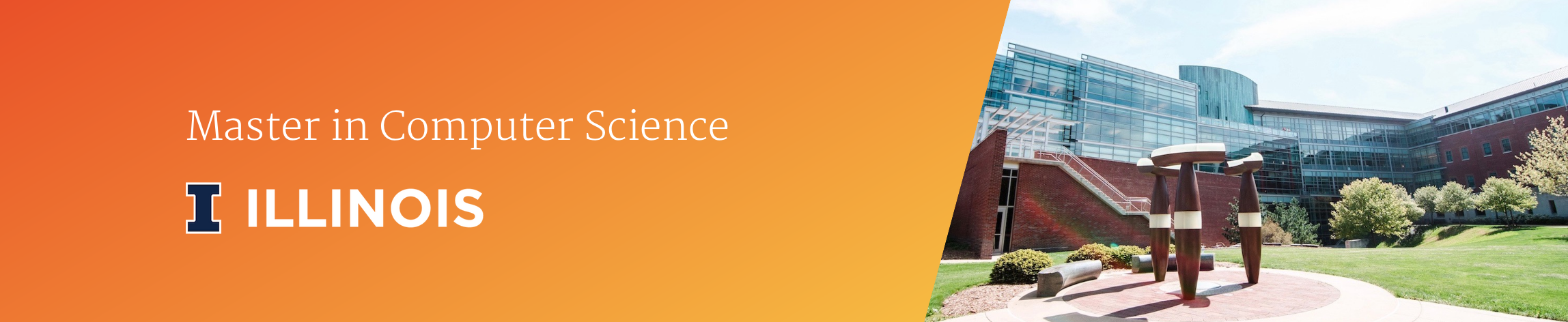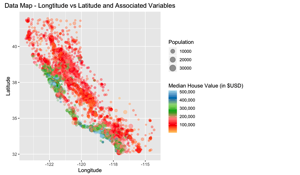I just completed Stat 420: Statistical Modeling in R. This course was extremely challenging over the summer (while also taking data visualization). With 10 homeworks and 10 quizzed and 2 exams in 12 weeks, there was always something due. In this class we covered a number of topics including linear regression and logistic regression. All of this course was done in the R programming language.

### Linear Regression ClassIn our group project we used the 1990 California Housing Data Set to work create a predictive model.

View my work here:

Homeworks for this class consisted of:

1. Data, Statistics, and R
2. Simple Linear Regression
3. Inference for Simple Linear Regression
4. Multiple Linear Regression
5. Categorical Predictors and Interactions
6. Diagnostics and Transformations
7. Collinearity and Model Selection
8. Regression for a Binary Response

Certificate